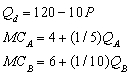# [Solved] Questions 94 Through 98 Refer to the Following

Question 94
Multiple Choice

## Questions 94 through 98 refer to the following. A firm with two plants, A and B, has the following estimated demand and marginal cost functions:-What is the firm's marginal revenue function?

A) MR = 12 (1/5)P
B) MR = 12 (1/5)Q
C) MR = 120 - 20P
D) MR = 120 20Q
E) none of the above

10+ million students use Quizplus to study and prepare for their homework, quizzes and exams through 20m+ questions in 300k quizzes.

Explore our library and get Economics Homework Help with various study sets and a huge amount of quizzes and questions

3.5K

Study sets

66.5K

Quizzes

5.9M

Questions

Upload material to get free accessInvite a friend and get free accessSubscribe and get an instant access